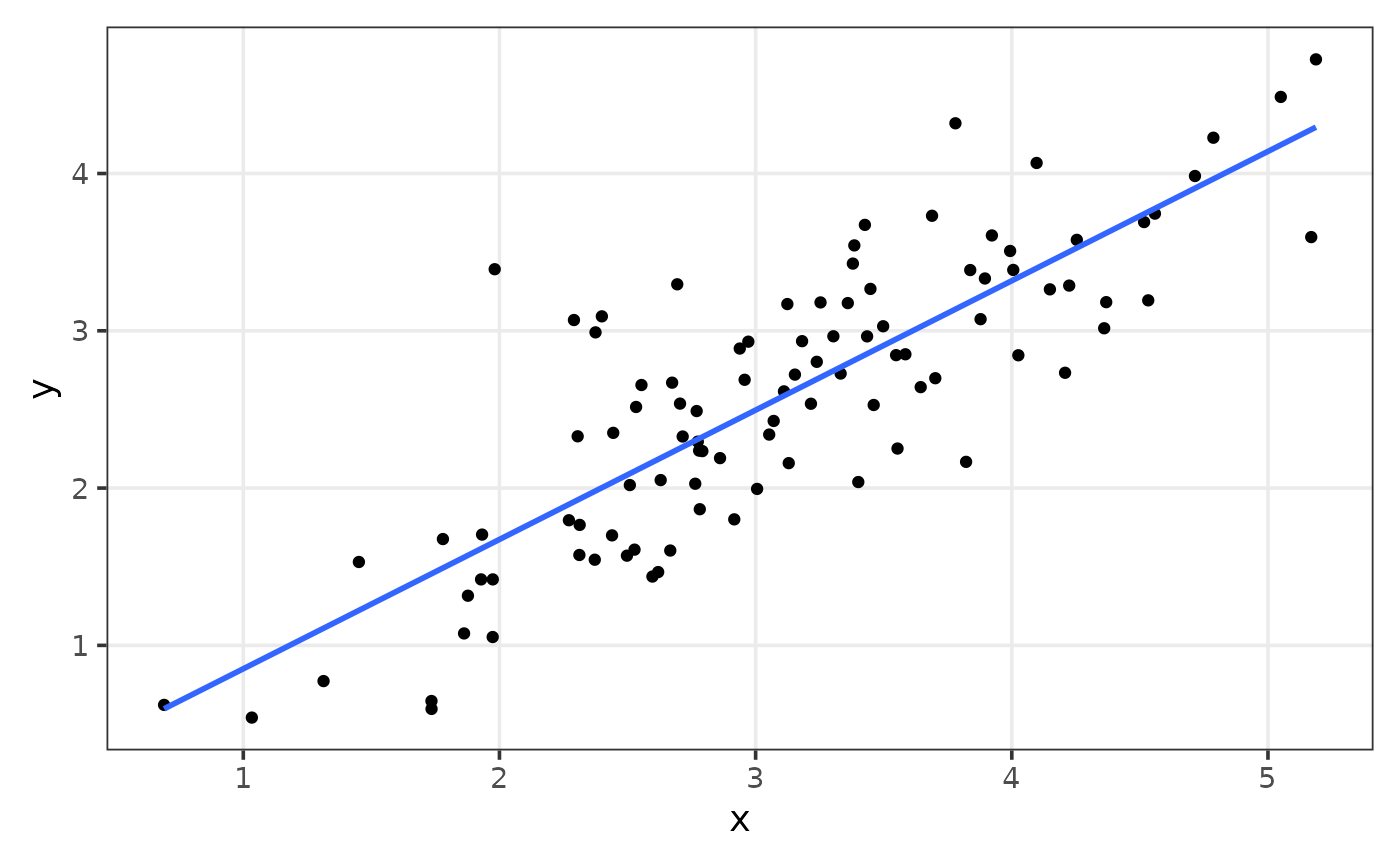library(relper)
library(dplyr)
library(ggplot2)

## cut_by_quantile

The goal of cut_by_quantile is to divide a numeric variable by a set of quantiles.


set.seed(123);x <- rnorm(100)

quartiles <- seq(0,1,by = .25)

table(cut_by_quantile(x,q = quartiles))
#>
#>  [-2.31,-0.494] (-0.494,0.0618]  (0.0618,0.692]    (0.692,2.19]
#>              25              25              25              25

## expand_grid_unique

The goal of expand_grid_unique is to create a grid of all combination from two variables, with no repetition of pairs, not matter the position.

expand_grid_unique(x = 1:3,y = 1:3)
#> # A tibble: 3 × 2
#>      V1    V2
#>   <int> <int>
#> 1     1     2
#> 2     1     3
#> 3     2     3

You can also set the argument include_equals to TRUE, so equal pairs are kept.

expand_grid_unique(x = 1:3,y = 1:3, include_equals = TRUE)
#> # A tibble: 6 × 2
#>      V1    V2
#>   <int> <int>
#> 1     1     1
#> 2     1     2
#> 3     1     3
#> 4     2     2
#> 5     2     3
#> 6     3     3

## obj_to_string

The goal of obj_to_string is to return the name of an R object as a string.

x <- c(1,2,3,5,7,8,12,100)

obj_to_string(x)
#>  "x"

## row_number_unique

The goal of row_number_unique is to get the row number, but considering the unique values of a variable.

tibble(x = c(1,1,1,2,3,4,5,5)) %>%
mutate(
row_number = row_number(),
row_number_unique = row_number_unique(x)
)
#> # A tibble: 8 × 3
#>       x row_number row_number_unique
#>   <dbl>      <int>             <int>
#> 1     1          1                 1
#> 2     1          2                 1
#> 3     1          3                 1
#> 4     2          4                 2
#> 5     3          5                 3
#> 6     4          6                 4
#> 7     5          7                 5
#> 8     5          8                 5

## rpearson

The goal of rpearson is to simulate data, where two variables will be linear correlated with a normal distribution, using pearson correlation coefficient as an argument.

set.seed(123);df <- rpearson(n = 100, pearson = .85, mean = 3)

df %>%
ggplot(aes(x,y))+
geom_point()+
geom_smooth(method = "lm", se = FALSE)+
plt_theme_xy()
#> geom_smooth() using formula = 'y ~ x'